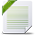# Decision Optimization

View Only

## Logical Constraint Modelling#### Archive UserWed October 16, 2019 04:25 PM#### ALEX FLEISCHERThu October 17, 2019 01:54 AM#### Archive UserThu October 17, 2019 04:20 AM#### Archive UserThu October 17, 2019 08:30 AM#### ALEX FLEISCHERThu October 17, 2019 09:11 AM#### Archive UserThu October 17, 2019 02:04 PM• #### 1.  Logical Constraint Modelling

Posted Wed October 16, 2019 04:25 PM

Originally posted by: memop

Hi.

I am trying to model some logical constraints -given below- in ILOG.

forall (j in tasks)  sum(i in stages) x[j,i] == 1;

forall (link in Linked)
Constraint_1:  sum (s in stages) s*x[link.linkedTask1][s] - sum(s in stages) s*x[link.linkedTask2][s]  == 0;

Constraint_2:  sum (s in stages) s*x[link.linkedTask1][s] - sum(s in stages) s*x[link.linkedTask2][2*m-s]  == 0;

forall (mind in MinDistances)
Constraint_3:  abs(sum (s in stages) s*x[mind.mindisTask1][s] - sum (s in stages) s*x[mind.mindisTask2][s]) >= mind.distance;

Constraint_4:  abs(sum (s in stages) s*x[mind.mindisTask1][s] - sum (s in stages) s*x[mind.mindisTask2][2*m-s]) >= mind.distance;

forall (maxd in MaxDistances)
Constraint_5:  abs(sum (s in stages) s*x[maxd.maxdisTask1][s] - sum (s in stages) s*x[maxd.maxdisTask2][s]) <= maxd.distance;
Constraint_6:  abs(sum (s in stages) s*x[maxd.maxdisTask1][s] - sum (s in stages) s*x[maxd.maxdisTask2][2*m-s]) <= maxd.distance;

Logical constraints could be given such as: Constraint_1 or Constraint_2, Constraint_3 or Constraint_4, Constraint_5 or Constraint_6. Only one of the constraints in each group should be satisfied and active, i.e if Constraint_1 is active, Constraint_2 should not be active, or vice versa.

I have tried some logical constraints definition method, e.g. Big M method, but I could not define the constraints and run the model. I would appreciate it if you have any suggestions. Thank you in advance. Regards.

• #### 2.  Re: Logical Constraint Modelling

Posted Thu October 17, 2019 01:54 AM

Hi,

could help

For xor you could write

dvar int x in 0..3;
dvar int y in 0..3;

subject to
{
(x==1) + (y==2) == 1;
}

regards

• #### 3.  Re: Logical Constraint Modelling

Posted Thu October 17, 2019 04:20 AM

Originally posted by: memop

Thank you Alex. I will try your suggestions.

Regards.

• #### 4.  Re: Logical Constraint Modelling

Posted Thu October 17, 2019 08:30 AM

Originally posted by: memop

Hi.

The model runs with these logical constraints approach. But I wonder, is it possible that the model could be established with mathematical formulation without these logical constraints?  As I said before, can any different formulation approach e.g. Big-M be used?

Regards.

• #### 5.  Re: Logical Constraint Modelling

Posted Thu October 17, 2019 09:11 AM

hi

you could have used bigM instead but logical constraints are much easier to write and read for human beings

regards

• #### 6.  Re: Logical Constraint Modelling

Posted Thu October 17, 2019 02:04 PM

Originally posted by: memop

Thank you Alex. I would like to try to establish the bigM model. However, since there are too many sum functions in my model, it is very challenging to build a big-m model. So I need your help.

Regards.

• #### 7.  Re: Logical Constraint Modelling

Posted Wed October 30, 2019 08:50 AM

Originally posted by: memop

Dear Alex, I have modeled my problem by using CPLEX (MIP Model) and CP (CP Model). The models run and all constraints are satisfied in two models. But both models produce different optimum solutions for the same data set. The models are attached to the post. Do I have a problem in modeling logical constraints? Thank you in advance.

Regards.

Attachment(s)CP Model.rar   2 KB 1 versionMIP Model.rar   2 KB 1 version